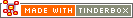^equal( data1, data2 )^Code Type: Boolean Comparison Code Scope of Action: item

^equal( data1, data2 )^

If data1 is equal to data2, this condition is true. to test inequality, test within ^not()^.

Result of `^equal(yes,yes)^` is: true

Result of `^equal(yes,no)^` is: false

 Up: Export Codes - Full Listing Previous: ^endsWith( target, data )^ Next: ^every( group , attribute[, data] )^

[Last updated: 14 Dec 2009, using v5.0]